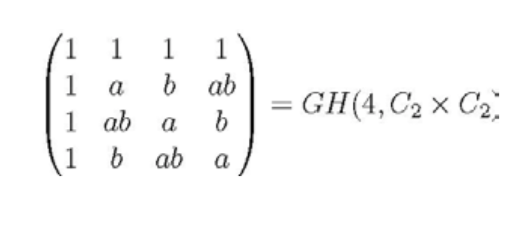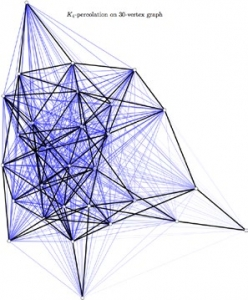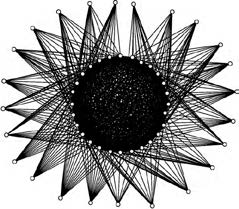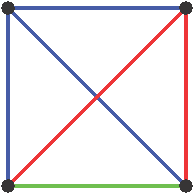## Research faculty members in this area

#### Combinatorial matrix theory

Combinatorial matrix theory studies the special properties of matrices subject to combinatorial restrictions such as that the entries must come from a certain set, or pairs of rows must be similar or dissimilar in specified ways. These objects model important things such as statistical experiments, codes for cryptological purposes or error correction in the presence of noise, and are used to design schemes for optical masking, filtering, telephone conferencing, radar, GPS and quantum cryptography. Key questions concern conditions for their existence, methods for their construction, their constituent structures and properties, and their connections to other fields of mathematics. One of the most celebrated unsolved problems of modern combinatorics is the Hadamard Matrix Conjecture.

Expertise in the Department also includes work on Hadamard matrices, weighing matrices, orthogonal designs, finite projective planes, as well as entry-wise nonnegative matrices.#### Extremal combinatorics Ramsey theory

Many questions in extremal combinatorics are of the form “How dense must a structure be before some property is guaranteed?”, and "What do the 'largest' structures look like that don’t have that property?" In graph theory, one may ask for the densest graphs that avoid a particular kind of subgraph. In arithmetic combinatorics, one might ask how many numbers from 1 to n are required before some arithmetic property is satisfied.

In Ramsey theory, one is concerned with the preservation of structure under partitioning or “colouring” of some small substructure (like an edge). The simplest cases in Ramsey theory follow from the pigeonhole principle. Extremal combinatorics and Ramsey theory are closely related; often a Ramsey-type question has an analogous formulation in extremal graph theory.

Tools used in both areas include graphs (and their generalizations), finite geometries, partial orders, topology, number theory, and the probabilistic method.

#### Random graphs

Random graphs are probability distributions on spaces of graphs and are studied to try to understand what sort of properties are typical or expected. The Erdős-Rényi random graphs are defined by taking a graph on some finite number of vertices with each possible edge included independently at random with some fixed probability. There are models of infinite random graphs like the family trees of Galton-Watson branching processes in which a random tree grows from a root with each vertex having a random number of child vertices independently according to a fixed distribution. Random graphs can also be defined by taking random subsets of a fixed (often large) graph.

Many properties of random graphs exhibit a threshold behaviour: very small changes in the value of some parameter lead to drastic changes in the likelihood of a graph having that property. Of interest is how processes on random graphs evolve over time. How does randomly scattered information spread in a graph? If some random subset of vertices are active and vertices become newly activated exactly when their number of activated neighbours is above some threshold, are all vertices eventually activated? How does a graph change when new edges are added to reinforce local connections? These questions are related to percolation and bootstrap percolation processes.#### Spectral graph theory

Spectral graph theory is the study of graph properties in terms of the eigenvalues and eigenvectors of certain matrices that are associated with a graph. Such matrices include the adjacency matrix, the Laplacian matrix, the signless Laplacian matrix, and the normalized Laplacian matrix. Each encodes the graph information in a different way, and the eigen-properties of these matrices reflect the structural properties of the graph such as connectivity and biparticity. Expertise in the Department in spectral graph theory includes work in all four of the types of matrices listed above and includes applications to food webs, protein interaction networks, and quantum walks on graphs.#### Graph invariants

A graph invariant is a property of a graph that is preserved by graph isomorphisms. The girth, the chromatic index, and the clique number are all examples of graph invariants. Current research revolves around investigating two novel graph invariants, the so-called minimum number of tile types and minimum number of bond-edge types, which have been introduced to develop optimal design strategies for certain DNA self-assembly processes. Questions of interest include determining relationships between these two invariants and classical graph parameters, as well as establishing methods to find their values for specific families of graphs. These problems are typically addressed using graph-theoretical tools, such as colorings and decompositions, and elementary linear algebra.